## Flipflop stationary state

Two MOSFET inverters are coupled to a flipflop, see Figure 1. The equations describe the stationary state. Because of the two identical inverters this problem has a natural symmetry, and we may expect bifurcation. In fact there is a bifurcation for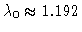, see Figure 2.

For the stationary states we have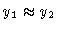and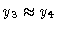. For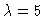there are three stationary states with y1=5, y3=0.16087 (stable) y1=y3=1.88577 (unstable) y1=0.16087, y3=5 (stable).

The dynamical behavior foris indicated in the phase diagram of Figure 3. Some trajectories are shown that approach the stable states.

The model is based on material kindly delivered by Siemens, München.

Figure 1
Two identical inverters coupled to a flipflop

Figure 2
Bifurcation diagram y1 versus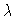.

Figure 3
(y1,y3) phase diagram for.

Figure 4
(y1,y3) phase diagram for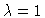.

This document
Figure 1
Figure 2
Figure 3
Figure 4